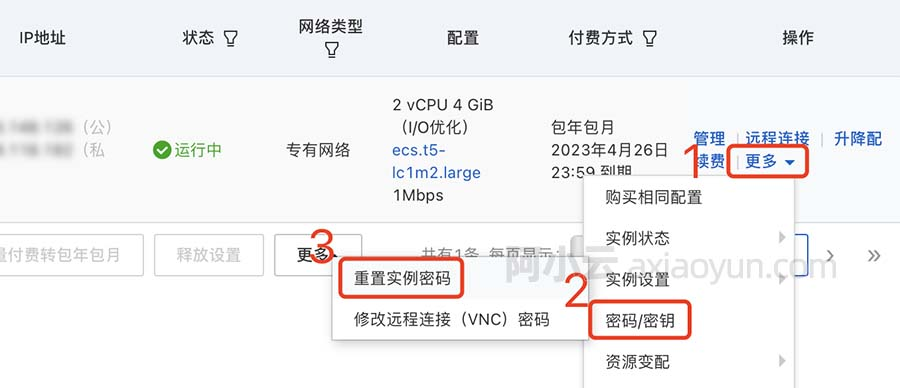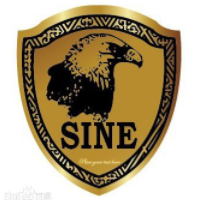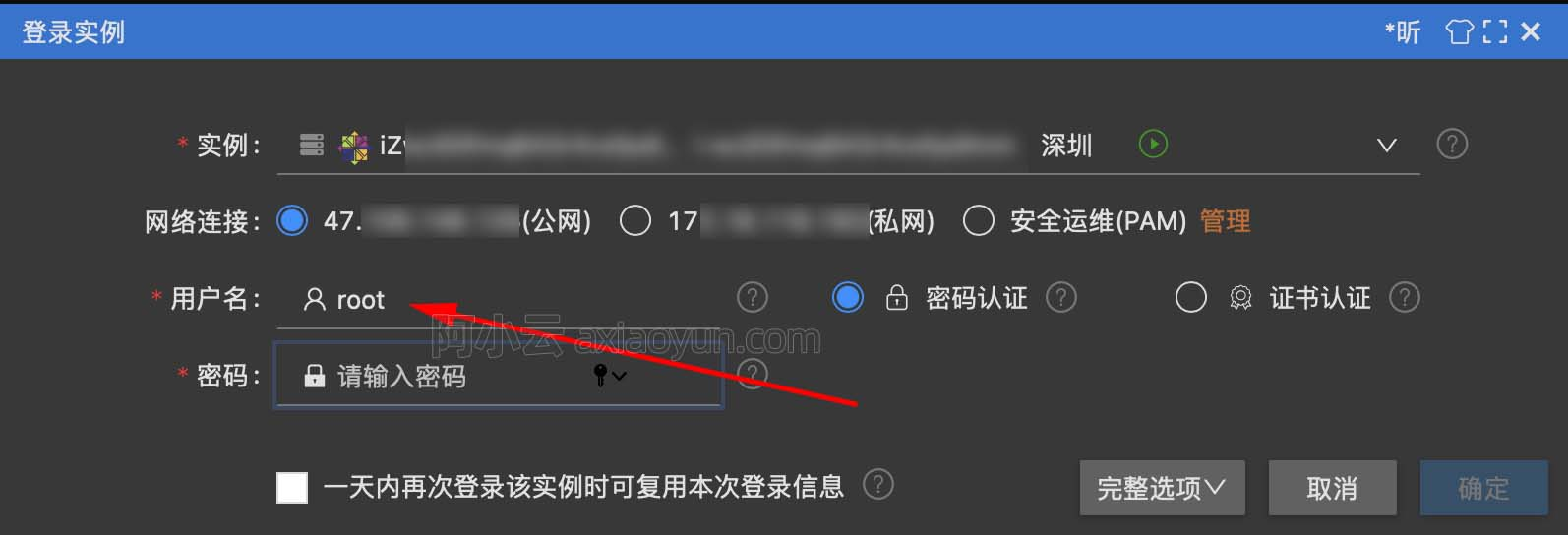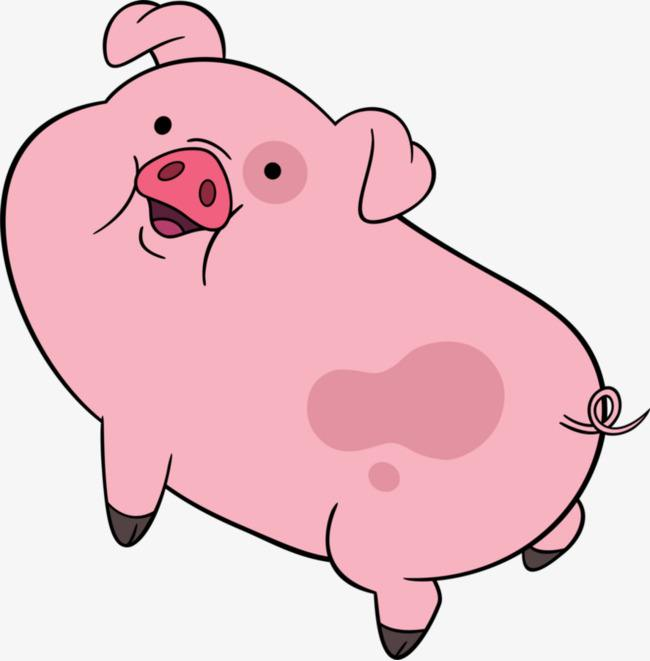【Go语言入门100题】023 输出GPLT (20 分) Go语言 | Golang

+关注继续查看

L1-023 输出GPLT (20 分) Go语言|Golang

pcTclnGloRgLrtLhgljkLhGFauPewSKgt

GPLTGPLTGLTGLGLL
• 1

package main

import "fmt"

func main() {
var str string
stdMap := make(map[rune]int)
_,_=fmt.Scan(&str)//GPLT
for _,item := range str { //map进行存储，key是字符，value是数字
if item == 'G' ||item == 'g' {
stdMap['G']+=1
}else if  item == 'P'||  item == 'p'{
stdMap['P']+=1
}else if item == 'L'||  item == 'l'{
stdMap['L']+=1
}else if item == 'T'||  item == 't'{
stdMap['T']+=1
}
}
s := ""
for {
if stdMap['G'] > 0 {  // 如果这个大于零
s+="G"   // 就让他输出
stdMap['G']-- // 然后让这个自减
}
if stdMap['P'] > 0 {
s+="P"
stdMap['P']--
}
if stdMap['L'] > 0 {
s+="L"
stdMap['L']--
}
if stdMap['T'] > 0 {
s+="T"
stdMap['T']--
}
if stdMap['G']==0 && stdMap['P']==0  && stdMap['L']==0 && stdMap['T']==0 {
break  // 如果全都没有的话，就直接退出了。
}
}
fmt.Printf("%s",s)
}9967 06728 014451 011572 0windows server 2008阿里云ECS服务器安全设置

9135 013727 022344 054708 07315 04444 0

157

0

《2021云上架构与运维峰会演讲合集》

《零基础CSS入门教程》

《零基础HTML入门教程》